## Mysql 查询一对多的两个表，多条数据全为相同的值

Xnzy 发布于 2018/03/01 11:28

为什么80%的码农都做不了架构师？->>>0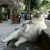X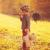select a.* from 教师表 a join 学生表 b on a.教师id=b.教师id where count(1)==sum(case b.性别 when '男' then 1 else 0 end )
X

0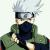X

X

X

0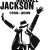select th.name from t_student stu,t_teacher th where stu.teacherid=th.id and stu.sex='男'

X

0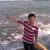X

0
X

0select t.* from (select a.id,count(1) count ,sum(case b.sex when '1' then 1 else 0 end) mc from js a join xs b on a.id=b.jsid GROUP BY a.id)t
where t.count=t.mc